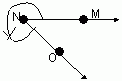Email us to get an instant 20% discount on highly effective K-12 Math & English kwizNET Programs!

#### Online Quiz (WorksheetABCD)

Questions Per Quiz = 2 4 6 8 10

### MEAP Preparation - Grade 5 Mathematics3.19 Classifying Angles

 Acute Angle: An angle whose measure is less than 90 degrees and greater then zero degrees is called an acute angle. 00 < m�A < 900 Right Angle: An angle whose measure is 90 degrees is called right angle. m�A = 900 Obtuse Angle: An angle whose measure is greater than 90 degrees and less than 180 degrees is called obtuse angle. 900 < m�A < 1800 Straight Angle: An angle whose measure is 180 degrees called a straight angle. m�A = 1800 Reflex Angle: An angle whose measure is greater than 180 degrees and less than 360 degrees is called a reflex angle. 1800 < m�A < 3600 Complete Angle: An angle whose measure is 360 degrees is called a complete angle. m�A = 3600 Zero Angle: If the measure of an angle is zero it is called a zero angle. m�A = 00 Directions: Answer the following questions. Also draw kinds of angles, measure them using a protractor and label them.
 Q 1: What is the type of the angle shown?Zero AngleComplete AngleStraight Angle Q 2: What is the type of the angle shown?Right AngleComplete AngleAcute angle Q 3: What is the type of the angle shown?Acute AngleObtuse AngleRight Angle Q 4: What is the type of the angle shown?Acute AngleReflex AngleRight angle Q 5: What is the type of the angle shown?Right AngleAcute AngleObtuse Angle Q 6: What is the type of the angle shown?Acute AngleComplete AngleRight Angle Question 7: This question is available to subscribers only! Question 8: This question is available to subscribers only!